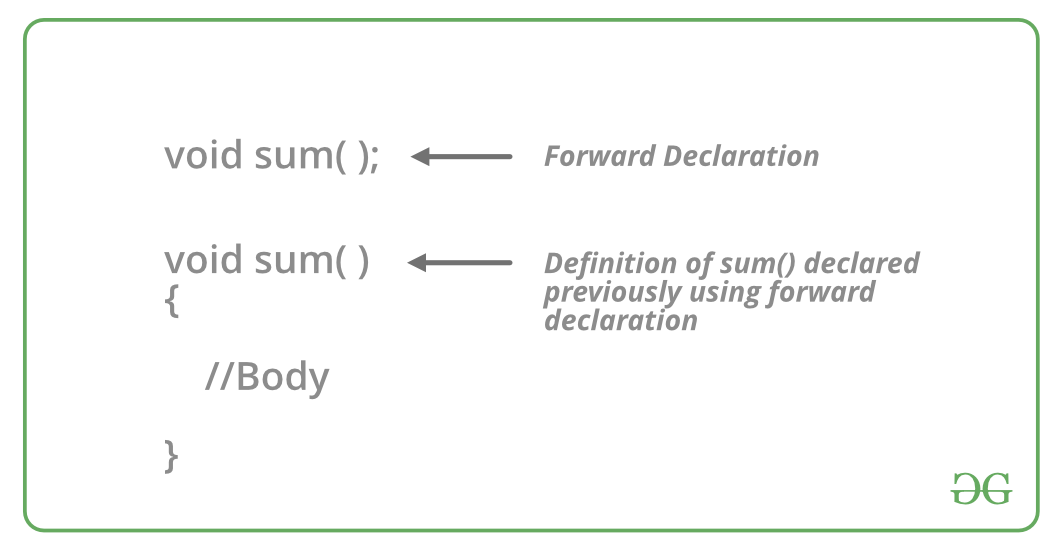# What are Forward declarations in C++

Forward Declaration refers to the beforehand declaration of the syntax or signature of an identifier, variable, function, class, etc. prior to its usage (done later in the program).Example:

```// Forward Declaration of the sum()
void sum(int, int);

// Usage of the sum
void sum(int a, int b)
{
// Body
}
```

In C++, Forward declarations are usually used for Classes. In this, the class is pre-defined before its use so that it can be called and used by other classes that are defined before this.

Example:

```// Forward Declaration class A
class A;

// Definition of class A
class A{
// Body
};
```

Need for Forward Declarations:

Let us understand the need for forward declaration with an example.

Example 1:

 `. ` `// C++ program to show ` `// the need for Forward Declaration ` ` `  `#include ` `    ``using` `namespace` `std; ` ` `  `class` `B { ` ` `  `public``: ` `    ``int` `x; ` ` `  `    ``void` `getdata(``int` `n) ` `    ``{ ` `        ``x = n; ` `    ``} ` `    ``friend` `int` `sum(A, B); ` `}; ` ` `  `class` `A { ` `public``: ` `    ``int` `y; ` ` `  `    ``void` `getdata(``int` `m) ` `    ``{ ` `        ``y = m; ` `    ``} ` `    ``friend` `int` `sum(A, B); ` `}; ` ` `  `int` `sum(A m, B n) ` `{ ` `    ``int` `result; ` `    ``result = m.y + n.x; ` `    ``return` `result; ` `} ` ` `  `int` `main() ` `{ ` `    ``B b; ` `    ``A a; ` `    ``a.getdata(5); ` `    ``b.getdata(4); ` `    ``cout << ``"The sum is : "` `<< sum(a, b); ` `    ``return` `0; ` `} `

Output:

```Compile Errors :
prog.cpp:14:18: error: 'A' has not been declared
friend int sum(A, B);
^
```

Explanation: Here the compiler throws this error because, in class B, the object of class A is being used, which has no declaration till that line. Hence compiler couldn’t find class A. So what if class A is written before class B? Let’s find out in the next example.

Example 2:

 `. ` `// C++ program to show ` `// the need for Forward Declaration ` ` `  `#include ` `    ``using` `namespace` `std; ` ` `  `class` `A { ` `public``: ` `    ``int` `y; ` ` `  `    ``void` `getdata(``int` `m) ` `    ``{ ` `        ``y = m; ` `    ``} ` `    ``friend` `int` `sum(A, B); ` `}; ` ` `  `class` `B { ` ` `  `public``: ` `    ``int` `x; ` ` `  `    ``void` `getdata(``int` `n) ` `    ``{ ` `        ``x = n; ` `    ``} ` `    ``friend` `int` `sum(A, B); ` `}; ` ` `  `int` `sum(A m, B n) ` `{ ` `    ``int` `result; ` `    ``result = m.y + n.x; ` `    ``return` `result; ` `} ` ` `  `int` `main() ` `{ ` `    ``B b; ` `    ``A a; ` `    ``a.getdata(5); ` `    ``b.getdata(4); ` `    ``cout << ``"The sum is : "` `<< sum(a, b); ` `    ``return` `0; ` `} `

Output:

```Compile Errors :
prog.cpp:16:23: error: 'B' has not been declared
friend int sum(A, B);
^
```

Explanation: Here the compiler throws this error because, in class A, the object of class B is being used, which has no declaration till that line. Hence compiler couldn’t find class B.

Now it is clear that any of the above codes wouldn’t work, no matter in which order the classes are written. Hence this problem needs a new solution- Forward Declaration.

Let us add the forward declaration to the above example and check the output again.

Example 3:

 `#include ` `using` `namespace` `std; ` ` `  `// Forward declaration ` `class` `A; ` `class` `B; ` ` `  `class` `B { ` `    ``int` `x; ` ` `  `public``: ` `    ``void` `getdata(``int` `n) ` `    ``{ ` `        ``x = n; ` `    ``} ` `    ``friend` `int` `sum(A, B); ` `}; ` ` `  `class` `A { ` `    ``int` `y; ` ` `  `public``: ` `    ``void` `getdata(``int` `m) ` `    ``{ ` `        ``y = m; ` `    ``} ` `    ``friend` `int` `sum(A, B); ` `}; ` `int` `sum(A m, B n) ` `{ ` `    ``int` `result; ` `    ``result = m.y + n.x; ` `    ``return` `result; ` `} ` ` `  `int` `main() ` `{ ` `    ``B b; ` `    ``A a; ` `    ``a.getdata(5); ` `    ``b.getdata(4); ` `    ``cout << ``"The sum is : "` `<< sum(a, b); ` `    ``return` `0; ` `} `

Output:

```The sum is : 9
```

The program runs without any errors now. A forward declaration tells the compiler about the existence of an entity before actually defining the entity. Forward declarations can also be used with other entity in C++, such as functions, variables and user-defined types.

My Personal Notes arrow_drop_upCheck out this Author's contributed articles.

If you like GeeksforGeeks and would like to contribute, you can also write an article using contribute.geeksforgeeks.org or mail your article to contribute@geeksforgeeks.org. See your article appearing on the GeeksforGeeks main page and help other Geeks.

Please Improve this article if you find anything incorrect by clicking on the "Improve Article" button below.

Article Tags :
Practice Tags :

4

Please write to us at contribute@geeksforgeeks.org to report any issue with the above content.